Trig Functions of Angles

a) Recall that the sine function is positive in Quadrants I and II, the cosine function is positive in Quadrants I and IV, and the tangent function is positive in Quadrants I and III.

b) In addition, the reference angle foris

1., if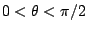.

2.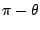, if.

3.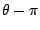, if.

4.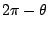, if.

c) Ifis an angle in standard position, andis a point on the terminal side of(other than the origin), then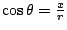andwhereEx 1 Find.

Sol The reference angle foris. Since the sine is negative in Quadrant IV,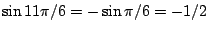.

Ex 2 Find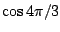.

Sol The reference angle foris. Since the cosine is negative in Quadrant III,.

Pr 1 Find.

Pr 2 Find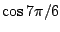.

Pr 3 Find.

Pr 4 Find.

Pr 5 Find.

Pr 6 Find.

Pr 7 Find.

Pr 8 Find.

Pr 9 Ifis an angle in standard position with the pointon its terminal side, find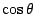and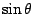.

Pr 10 Find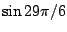.

Pr 11 Find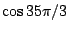.